# The Speed of Sound

NOTE

This manual describes the laboratory experiment used during the 1996 - 1997 academic year. Significant changes have been made since then, and the manual used during the current academic year is in NOT available yet on the WEB. Hardcopies can be purchased at the bookstore.

## Purpose

To determine the speed of sound in air and in metal.

## Introduction

Recall our knowledge of standing waves established in a stretched string which is fixed at both ends. A wave traveling down the length of the string is reflected at the end and returns in the opposite direction (with the same wavelength). If the length of the string is chosen such that the superposition of these two waves establishes a pattern of standing waves, we call this condition resonance.

Likewise, standing sound waves can be achieved in a tube with either one or both ends open. As with the stretched string, resonance is established if the length of the tube is chosen appropriately according to the wavelength (or frequency) of the sound waves. The open end of the tube corresponds to the location of a displacement antinode, i.e., where the amplitude of the oscillations is at its maximum value. The closed end of the tube corresponds a displacement node, i.e., where the amplitude of the oscillations is zero.

The fundamental harmonic corresponds to the lowest frequency at which standing waves are established. The second harmonic is the next highest frequency resulting in standing waves. The same applies for higher order harmonics.

As we know, the speed (v), the frequency (f), and the wavelength ([[lambda]]) are related by

v = [[lambda]] f (11.1)

Thus, if we can determine the wavelength ([[lambda]]) of sound within a tube and its frequency (f), then we can easily determine the speed of sound (v).

## Prelab Homework

The following homework must be finished prior to class and should be turned in to the lab TA before the start of the lab. Also, please read the instructions for this lab.

### Questions

• What is the longest wavelength at which a sound wave will be resonant in a pipe of length L which is open at one end and closed at the other? Assume that for resonance there will be an anti-node in the displacement of the air at the open end and a node at the closed end.

• Derive a formula which will give all the allowed (resonant) wavelengths for such a pipe in terms of its length L.

## The experiment

### Part I: Speed of Sound in Air

Longitudinal vibrations produced by a tuning fork are transmitted through the air into a plastic tube with an adjustable piston at one end (see the Figure 11.1). By suitably adjusting the length of the air column using the piston, standing waves are produced in it. The locations of the nodes of the standing waves are determined by the lengths of the air column required to produce resonance. By knowing the distance between the nodes, the wavelength of the vibration can be found. Since the frequency of the tuning fork is given (it is stamped on the side of the fork), the speed of sound in the air can be calculated.

Let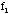be the frequency of vibrations of the tuning fork. This will also be the frequency of the tone in the air column. If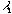is the wavelength of the sound in air, then the speed of sound in air is just,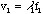(11.2)

#### Procedure

• Set up the apparatus as shown in the diagram below. Position the tuning fork at the end of the plastic tube with its edge about 1 cm from the open end of the tube.Figure 11.1

• Firmly strike the tuning fork with the rubber mallet. Be careful that the tuning fork does not strike the tube.

• Vary the effective length of the tube by moving the piston through its complete range and determine the positions of each of the nodes by listening for resonance. Loud resonance corresponds to an antinode (also found at the open end of the tube).

• Record the distance of each node from the open end of the tube, and make an estimate of the uncertainty in the measurements.

• Record the frequency of the tuning fork.

• Repeat previous steps with at least two other tuning forks of different frequencies.

### Part II: Speed of Sound in a Metal Rod

To determine the speed of sound in a metal rod, we will use a procedure known as Kunzt's method. Longitudinal vibrations, produced in a uniform metal rod clamped at its center, are transmitted to the air inside a tube closed at one end (see Figure 11.2). By adjusting the effective length of the air column, standing waves are produced. The presence of the standing waves is determined in this case by placing some light powder or dust in the tube. The vibration of the air causes the dust to move about. Consequently the dust tends to move from where the amplitude of the air vibrations is large to where it is small. That is, the dust collects into heaps at the nodes. Thus, the wavelength of the standing waves in air is determined from the separation of the dust heaps. Using the speed of sound in the air determined in Part I, the frequency of the vibrations can be obtained. The speed of sound in the rod is calculated using the length of the rod and the frequency of the vibrations. For this experiment the length of the rod is equal to 1/2 of a wavelength of sound (within the rod). We will use an aluminum rod, in which the speed of sound is approximately 5100 m/s at room temperature.

#### Procedure

• Deposit a thin layer of lycopodium powder at least 2 mm wide as uniformly as possible inside the plastic tube. The apparatus should then be set up as shown below. Be sure to clamp the metal rod at its center and position the plastic tube so that the rod is centered vertically within the tube without touching the tube.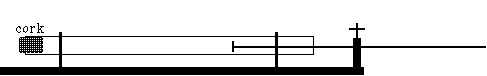Figure 11.2

• Set the rod in vibration by stroking it slowly, from the center to the free end, with a cloth or chamois wet with alcohol or rubbed with rosin. Make sure the rod does not touch the tube. You should hear a high-pitched squeaking sound.

• Adjust the effective length of the air column in the tube (by moving the tube horizontally) until wave patterns are formed in the dust. A slight rotation of the plastic tube often establishes an easily measurable pattern.

• Measure the distance between consecutive piles of dust, taking note also the uncertainty of the measurement.

Let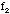be the frequency of the vibration produced in the rod. This will also be the frequency of the corresponding vibrations in the air. Let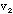be the speed of sound in the rod, [[lambda]]2 be the wavelength of sound within the rod, andcorrespond to wavelength of the vibration in the air. We then have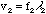(11.3)

and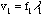(11.4)

But, since, dividing(11.3) by (11.4) gives,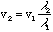(11.5)

With the rod clamped at its center point, the length of the rod L is a half wavelength of the sound in the rod, or equivalently,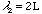. Thus,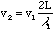(11.6)

## Data Analysis

### Part I:

• Calculate the distance between consecutive nodes. An accurate measurement of this distance is to measure the distance between n nodes and then divide by (n-1).

• Calculate the speed of sound in air for each of the measurements you made. Determine the uncertainty and average value.

• Compare your results with the value resulting from the following formula for the speed of sound in air as a function of temperature,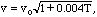(11.2)

where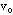= 332 m/sec is the speed of sound in air at 0 deg.C and T is the temperature in degrees Celsius.

### Part II:

• Describe the behavior of the lycopodium powder. Where are the nodes? Where are the anti-nodes?

• Using your measured value for the speed of sound in air (from Part I) and your data from Part II, determine the speed of sound in the metal rod, and the uncertainty in the calculated value. Compare with known values of the speed of sound in the metal of which the rod is composed. (Again, for greater accuracy measure the distance of n piles.)

### Questions

• Explain why the frequency of the sound does not change as the sound waves go from the rod to the air. If the frequency does not change, why does the wavelength change? Give some other examples of vibration or wave motion where this occurs.

• Explain why the length of the rod is half a wavelength. Make a sketch of the rod showing the amplitude of the sound wave in the rod. Mark the anti-node(s) with "A" and the node(s) with "N." On the same sketch indicate the physical motion of the rod along its length.

• In Part II of the experiment, if one end of the tube had been left open instead of being closed, could standing waves have been set up by adjusting the length of the tube? If so, would the internodal distances have been changed? Explain.

Send comments, questions and/or suggestions via email to wolfs@nsrl.rochester.edu.